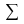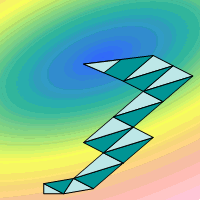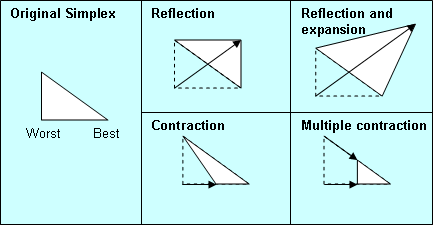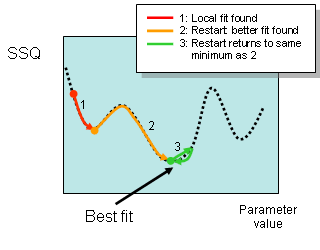Scale-up Suite Help
Set fitting algorithm & tolerance
Accessing fitting algorithm controls

Advanced users can change the default algorithm settings, but we recommend the default settings for everyday use.

To view the algorithm settings do one of the following:

• click the Advanced Options iconin the Fitting toolbar.

You can also change Simulator run parameters in this dialog box.

The Simplex algorithm is also available for fitting exercises via the Fitting Algorithm dropdown. It is a simple, robust and widely used method. It is useful for noisy data, but can be slow, especially when fitting a large number of parameters.More on the Simplex algorithm

A Simplex is a geometrical figure. For fitting or optimization each vertex represents a different set of parameter values. The number of vertices in the Simplex is one more than the number of parameters being varied (e.g. if you select two parameters, the Simplex is a triangle (3 vertices), for 3 parameters it is a tetrahedron (4 vertices)). After each iteration, the worst-fitting point is discarded and the Simplex is modified. Four operations are permitted on the Simplex to improve the fit: reflection, reflection with expansion, contraction and multiple contraction. Examples are shown below for a two parameter case.DynoChem uses combinations of these in a sequence, which adapt to each model in order to find the best fit possible.
Setting Tolerance

This refers to the termination criterion used by the fitting algorithm and determines when the run is considered complete. A small number means that, in general, more iterations will be performed before judging that a minimum has been found. Values of 0.01 through to 1e-8 are available in the Tolerance dropdown.

Automatic Restarts

Automatic Restarts provide an in-built sensitivity check on the fit obtained. If 'restarts' are On the fitting exercise will be re-run twice on completion (i.e. a total of three times). The values from the previous run form part of the new set of initial guesses for the algorithm. In each restart, Dynochem attempts to find a better fit using significantly different initial guesses. The purpose of this feature is to test for a local minimum, i.e. to test whether the fitted parameter values are independent of the initial guesses and that the true global minimum has been located.Set the maximum number of iterations

The default number of iterations for the fitting algorithm is 200 and can be changed by typing in the Max iterations input box. In many cases, the actual number required for a fit will be significantly lower than this. At each iteration a new Simplex is generated that is better than the previous one.